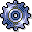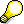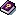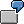The Cost Center Accounting/Asset Accounting InterfaceUse

In integrated planning between the Cost Center Accounting and Asset Accounting, you can transfer depreciation and interest for an asset (calculated periodically) to primary cost planning for Cost Center Accounting.If you want to transfer depreciation and interest from planned investments for asset acquisitions in the fiscal year to cost center planning, you need to note the following:

• You can plan costs that cannot be activated or receive interest (as well as those that can) on the objects in investment planning.
• The SAP R/3 System calculates depreciation and interest using the overall plan value.
• To restrict depreciation and interest planning for planned investments on the true portion of investments that can be activated, enter a flat-rate percentage for each valuation area. Enter this percentage for the expected portion of the planned overall costs that can be activated.

Integration

If you record assets in different company codes for the same cost center, then the system transfers only the value of one company code when it transfers the planned depreciations to cost center planning. To ensure a complete transfer, you can do the following:

• You can set up your own G/L accounts and cost elements for the assets whose depreciation is to be transferred to cost centers in other company codes.
• For each cost center and company code, you can create a plan-integrated order that is settled to each cost center. You assign the affected assets to each order, so that the depreciation on this order can be planned and posted.
• If only a few cost centers are affected, you can also plan the depreciation manually.
• You should also take into account the structure of your cost accounting, as well as the assignment of the assets to cost centers. A cost center must be uniquely assigned to a company code, also in cost accounting which is cross-company code.

Prerequisites

To transfer data from Asset Accounting to Cost Center Accounting, proceed as follows:

1. Define an asset class
2. In the asset class you define the assignment for all assets that are assigned to this class by specifying an existing cost center and an activity type for activity-dependent planning. The assets class is linked to a chart of depreciation which you have created. It contains a number of different depreciation areas designed to handle depreciation for a particular purpose (such as book or tax depreciation).

3. Create valid asset master records
4. The SAP R/3 System posts the depreciation and interest based on the chart of depreciation defined in the asset class. You can transfer these values to activity-independent primary cost planning in Cost Center Accounting.

5. Store the cost center/activity type in the asset master data record

For more information about primary cost planning in Asset Accounting, seeBasic Functions for Organizational Structures.

The following conditions must apply in Cost Center Accounting:

1. You have created valid cost centers
2. Valid cost elements
3. If you want to transfer activity-independent values, you need to define valid activity types and carry out activity type planning.
4. You have defined valid planner profiles and planning layouts.

5. You have created a version (if you are not planning with version 000).
6. You have defined distribution keys (if you are not using standard distribution keys)In controlling area 0001 you have created fixed asset 15010, assigned to asset class 1000/chart of depreciation 1DE. On 15 April 1994 you post an external acquisition of \$20,000 to this asset. The SAP R/3 System calculates the depreciation and interest for the asset according to the depreciation areas defined in the chart of depreciation. In this example this value is \$2000/year (straight-line depreciation over ten years).

You can transfer this value to primary cost planning in cost center accounting. If you entered distribution key 01, the SAP R/3 System distributes the \$2000 equally to all the periods in the current fiscal year.

 Period Depreciation Value 01 2000/12 02 2000/12 . . . 12 2000/12 Total 2000